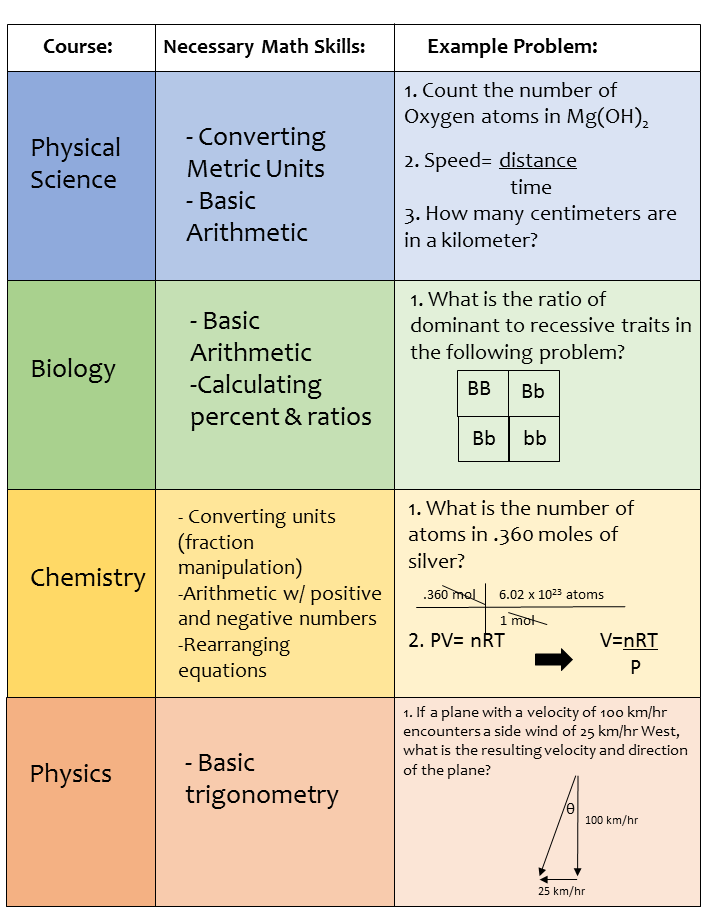Search
•Anne Morgan

# A Beginner's Guide to Math and Science: How to Know if Your Child Is Ready

"Mathematics is a part of science...a set of tools that helps scientists understand a marvelously complex world." -Dr. Paul Williams, Biology Professor at Univ. of Wisconsin

If my child is only halfway through Algebra 1, can she take Chemistry?  What science course should my son take as a 9th grader? Most parents understand that math and science are connected, but still struggle to determine which math skills are required for each science course.The answers to these questions are obviously dependent on your child's strengths, weaknesses, post-graduation plans, and the requirements of your state.  However, there are some guidelines that may be helpful to you as you plan a high school math and science sequence.  And since it's likely been a long time since your high school days (sad, but true), you might not remember enough details about your science courses to make these decisions without some research.

There are some guidelines that may be helpful to you as you plan a high school math and science sequence.

Although it's also been a disturbingly long time since my high school days, I have taught high school science for many years.  Because I've seen this question arise time and time again in online homeschooling communities, I thought I'd provide some assistance.As you plan for the high school years, keep in mind the following skills required for each of the following science courses:

## 1. Physical Science:

Physical Science is not a math-heavy course, which is what makes it perfect for the introductory high school years, or even later middle school years.  A solid understanding of basic arithmetic and metric units is all that's really required for this course.  Students may need to convert centimeters to kilometers, or use "plug and chug" equations, in which they put units into a given formula such as Speed= distance/time.  Sometimes, the equation may need to be rearranged in order to solve for a different variable, which requires some basic algebra skills, but teachers and textbooks rarely assume that students taking physical science can do this automatically.  The steps to solve these equations are usually laid out clearly.

## 2. Biology

Biology is likely the least math-intensive science field.  At least in the high school years, biology simply requires an understanding of basic arithmetic.  Students may be asked to know how atoms form molecules, which sometimes involves addition and subtraction of exponents.  They will certainly be required to have a solid understanding of measuring in the metric system, as well as converting between metric units.  Genetics and heredity also involve some statistics, but this rarely goes beyond calculation of ratios and percents, unless students are taking an advanced version of Biology.

(If Biology is in your high school plans, please take a second to see if our Biology curriculum matches with your child's learning style.)

## 3. Chemistry

Chemistry is the first science course that is heavily dependent on math skills.  Students without a very solid foundation in algebra will consistently struggle in a traditional chemistry course.  One of the over-arching themes in chemistry involves the conversion and canceling of units, which is called dimensional analysis.  This process works the same way as multiplying and dividing fractions, but students are required to manipulate factors in order to cancel out units.  Because students need to set up the steps on their own, they should be able to reduce, multiply, and divide fractions without much thought or they will get bogged down in the math and have an even more difficult time understanding the underlying chemistry concepts.

Many mathematical equations are provided in chemistry and students will need to manipulate and rearrange the equations to solve for a specific variable.  If this is not second-nature, students will become increasingly frustrated with chemistry.

Students will also be asked to balance molecular equations in chemistry, which requires skills in factoring, adding and subtracting exponents, and solving for variables.  If your child is taking a traditional chemistry course, she should have at least Algebra 1 completed, or frustration is sure to follow.

## 4. Physics

Math is the language of physics.  Students taking a traditional physics curriculum will regularly use the Pythagorean theorem, the quadratic equation, geometry of two-dimensional and three-dimensional shapes, the trigonometric functions of sin, cos, and tan, as well as combining equations to solve for one or multiple variables.  Students must have a solid foundation in algebra, geometry, and either have completed or be simultaneously taking calculus or algebra 2/trigonometry.

In addition to these course-specific requirements, your child should also have a fundamental understanding of how to make and read graphs and charts of all kinds.  These skills will be of infinite value if the ACT is in your future plans, as well.

What about "math-free" versions of Chemistry or Physics?These are obviously only general guidelines, and you know your child best.  However, I urge you to consider your child's math abilities as you choose a science curriculum.  Because these two fields are so closely linked, without a solid math foundation, students can become frustrated and miss out on the enjoyment that can come from science courses.

Parents also often ask if their child should take a "math-free" version of Chemistry or Physics.  Unless your child is 100% sure that they will not be entering a science or mathematics field, I would strongly caution against this.  I know it seems like the easiest option when you have a high school student that's fighting you at every turn, but please keep in mind that there are consequences for choosing the easiest option.  Students often change their minds about college majors, and if your child does decide to enter a STEM field of any kind, he will be at a serious disadvantage among his undergraduate peers.